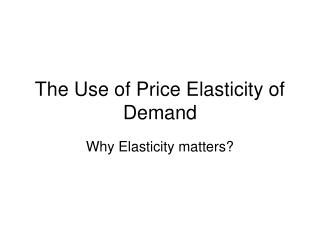DownloadDownload PresentationThe Use of Price Elasticity of Demand

# The Use of Price Elasticity of Demand

Télécharger la présentation## The Use of Price Elasticity of Demand

- - - - - - - - - - - - - - - - - - - - - - - - - - - E N D - - - - - - - - - - - - - - - - - - - - - - - - - - -
##### Presentation Transcript

1. The Use of Price Elasticity of Demand Why Elasticity matters?

2. Elasticity, Total Revenue, and Demand • The elasticity of demand tells suppliers how their total revenue will change if their price changes. • Total revenue equals total quantity sold multiplied by price of good.

3. Elasticity, Total Revenue, and Demand • If ED is elastic (ED > 1), a rise in price lowers total revenue. • Price and total revenue move in opposite directions.

4. Elasticity, Total Revenue, and Demand • If ED is unit elastic (ED = 1), a rise in price leaves total revenue unchanged.

5. Elasticity, Total Revenue, and Demand • If ED is inelastic (ED< 1), a rise in price increases total revenue. • Price and total revenue move in the same direction.

6. \$10 8 F Gained revenue 6 Price Lost revenue 4 2 0 1 2 3 4 5 6 7 8 9 Quantity Elasticity and Total Revenue Unit Elastic Demand E = 1 TRE= \$4x6=\$24 TRF= \$6x4=\$24 TR constant C E A B

7. Gained revenue Lost revenue Elasticity and Total Revenue Inelastic Demand E < 1 \$10 TR rises if price increases 8 TRG = \$1 x 9 = \$9 TRH = \$2 x 8 = \$16 6 Price 4 H 2 G C A B 0 8 1 2 3 4 5 6 7 9 Quantity

8. Gained revenue J K Lost revenue B Elasticity and Total Revenue Elastic Demand E > 1 \$10 C TR falls if price increases. 8 TRJ = \$8 x 2 = \$16 TRK = \$9 x 1 = \$9 6 A Price 4 2 0 1 2 3 4 5 6 7 8 9 Quantity

9. Total Revenue Along a Demand Curve • With elastic demand – a rise in price lowers total revenue. • With inelastic demand – a rise in price increases total revenue.

10. Q0 Price 0 Quantity Q0 Total Revenue Along a Demand Curve Elastic ED > 1 ED = 1 Inelastic ED< 1 Total revenue 0 Quantity

11. Elasticity of Individual and Market Demand • Price discrimination occurs when a firm separates the people with less elastic demand from those with more elastic demand.

12. Elasticity of Individual and Market Demand • Firms that price discriminate charge more to the individuals with inelastic demand and less to individuals with elastic demands.

13. Elasticity of Individual and Market Demand • Examples of price discrimination include: • Airlines’ Saturday stay-over specials. • The phenomenon of selling new cars. • The almost-continual-sale phenomenon.